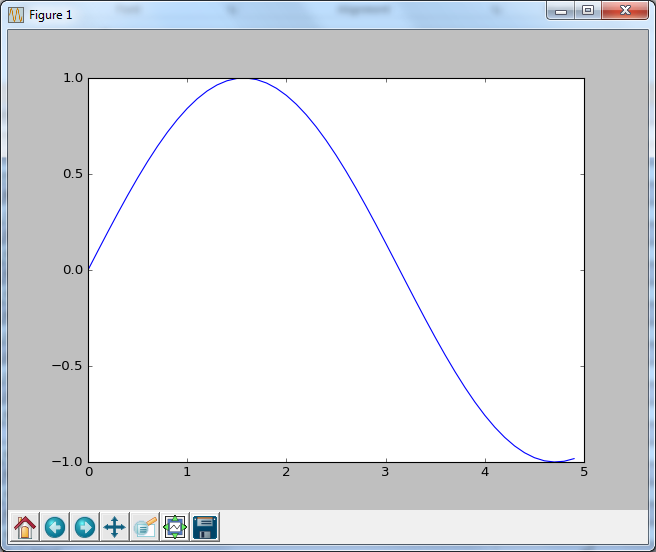Python  - Matplotlib                                                       Home : www.sharetechnote.com

Matplotlib

One of the feature that I thought of as the limitation of Python was lack of graphics/plot function. However, even if it is not a part of basic installation, you can add very powerful graphics/plot functionality like Matlab by installing a special library called matplotlib. You can get the library from following location.

Official Site :  http://matplotlib.org/

Installation

You can download and install the package from the above link, or install using pip as shown below. Definately I prefer pip method.

c:\Python35>pip install matplotlib

Collecting matplotlib

100% |################################| 6.2MB 178kB/s

Requirement already satisfied: numpy>=1.6 in c:\python35\lib\site-packages (from

matplotlib)

Requirement already satisfied: python-dateutil in c:\python35\lib\site-packages

(from matplotlib)

Collecting pyparsing!=2.0.4,!=2.1.2,>=1.5.6 (from matplotlib)

100% |################################| 61kB 5.1MB/s

Collecting cycler (from matplotlib)

Requirement already satisfied: pytz in c:\python35\lib\site-packages (from matpl

otlib)

Requirement already satisfied: six>=1.5 in c:\python35\lib\site-packages (from p

ython-dateutil->matplotlib)

Installing collected packages: pyparsing, cycler, matplotlib

Successfully installed cycler-0.10.0 matplotlib-1.5.3 pyparsing-2.1.10

Basic Check

Once you installed the package, try quick check whether it is properly installed or not. You can simply type in several lines of commands as shown below.

>>> import numpy as np

>>> import matplotlib.pyplot as plt

>>> x = np.arange(0, 5, 0.1);

>>> y = np.sin(x);

>>> plt.plot(x, y)

[<matplotlib.lines.Line2D object at 0x05D61FB0>]

>>> plt.show()or create a file as below and run.

 matplot_BasicCheck.py import numpy as np import matplotlib.pyplot as plt   x = np.arange(0, 5, 0.1); y = np.sin(x); plt.plot(x, y) plt.show()

NOTE : Matplotlib functionality and concept is very similar to the plotting in Matlab. If you are familiar with Matlab plot functions, you would catch up Matplotlib functions very easily.

Reference :